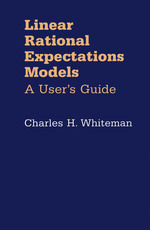Linear Rational Expectations Models: A User’s Guide

Charles H. Whiteman
Edition: NED - New edition
https://doi.org/10.5749/j.ctttsf26
Pages: 152
https://www.jstor.org/stable/10.5749/j.ctttsf26

Export Selected Citations Export to NoodleTools Export to RefWorks Export to EasyBib Export a RIS file (For EndNote, ProCite, Reference Manager, Zotero, Mendeley...) Export a Text file (For BibTex)
1. Front Matter
(pp. i-vi)
2. Preface
(pp. vii-x)
Charles H. Whiteman
(pp. xi-xiv)
4. I Moving Average Representations for Scalar Rational Expectations Models
(pp. 1-36)

Although superficially similar, the equations to be studied in this book are quite unlike ordinary stochastic difference equations: each contains a term involving an expectation. For this reason, the objects to be studied are referred to as “expectational difference equations.” This nomenclature distinguishes the objects of study from ordinary stochastic difference equations in a way that Shiller’s (1978) term “linear difference models” does not.

The simplest example of an expectational difference equation is

(1)${{\rm{E}}_{\rm{t}}}{{\rm{y}}_{{\rm{t}} + 1}} - \rho {{\rm{y}}_{\rm{t}}} = {{\rm{x}}_{\rm{t}}}.\quad \rho \;\varepsilon \;{\rm{R,}}\;\rho \; \ne \;0$

For most purposes, t indexes the integers, and${{\rm{x}}_{\rm{t}}}\;{\rm{and}}\;{{\rm{y}}_{\rm{t}}}$may be thought of as representative elements in sequences of random variables. The variable${{\rm{x}}_{\rm{t}}}$is...

5. II Solution Techniques for Rational Expectations Models: A Critical Review
(pp. 37-70)

If one reads between the lines of the recent rational expectations literature, one can find there an implicit controversy awaiting transformation into an explicit one. The unstated issue concerns the efficacy of various algorithms for finding reduced forms in rational expectations models. Such models are, for the most part, systems of linear stochastic difference equations in which agents’ views about the future enter in a fundamental way. If these expectations are formed adaptively, using fixed linear functions of available information, the problem of finding a reduced form is equivalent to finding the solution to a standard difference equation. The techniques...

6. III Confronting Nonuniqueness in Rational Expectations Models
(pp. 71-89)

Some rational expectations models possess more than one solution. This should come as no surprise, for most of the rational expectations models which have appeared to date are, in essence, difference equations without boundary conditions.¹ There are, however, different types of nonuniqueness. One stems from the self-fulfilling nature of rational expectations. Another arises only for certain values of the parameters of the model.

In two recent papers, Taylor (1977) and McCallum (1980) propose alternative methods for dealing with nonuniqueness. Taylor’s method, the “minimum variance” condition, chooses the solution with the smallest variance, while McCallum’s “minimum state variable” condition chooses the...

7. IV Moving Average Representations for Multivariate Rational Expectations Models
(pp. 90-109)

Methods for solving multivariate rational expectations models have recently been studied by, among others, Blanchard and Kahn (1980), Chow (1981), Fair and Taylor (1980), and Futia (1981). But their procedures do not generally provide the solution in a form convenient for, say, estimation. The analysis herein exploits the properties of polynomial matrices to establish conditions for the existence and uniqueness of solutions to multivariate linear rational expectations models. But more important, a method similar to the one employed in Chapter I is utilized to find “closed form” expressions for solutions when they exist. Furthermore, the method used here is suggestive....

8. V A set of Optimization Examples
(pp. 110-124)

One of the advantages of the technique studied in previous chapters is that it can be used to solve a wide class of rational expectations models. The technique is useful whether the expectational difference equation is viewed as the primitive objective of the model or as the first-order condition of some fully specified optimization problem. The purpose of this chapter is to investigate this latter claim; to show how expectational difference equations arise as necessary conditions for equilibria in a class of dynamic equilibrium models, and to show how the equilibria can be calculated using the techniques decribed in Chapters...

9. References
(pp. 125-129)
10. Author Index
(pp. 130-131)
11. Subject Index
(pp. 132-134)
12. Back Matter
(pp. 135-135)